## RS Aggarwal Class 8 Solutions Chapter 3 Squares and Square Roots Ex 3A

These Solutions are part of RS Aggarwal Solutions Class 8. Here we have given RS Aggarwal Solutions Class 8 Chapter 3 Squares and Square Roots Ex 3A.

Other Exercises

Question 1.
Solution:
(i) 441
= 3 x 3 x 7 x 7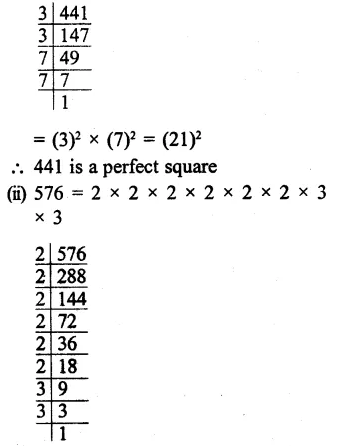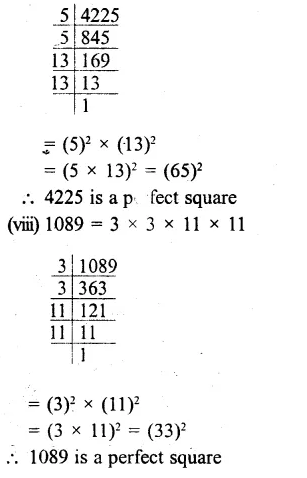Question 2.
Solution:
(i) 1225
= 5 x 5 x 7 x 7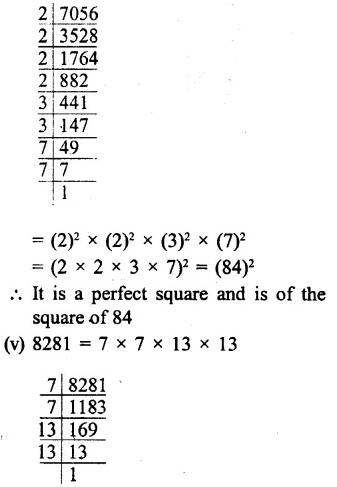Question 3.
Solution:
(i) Factors of 3675
3 x 5 x 5 x 7 x 7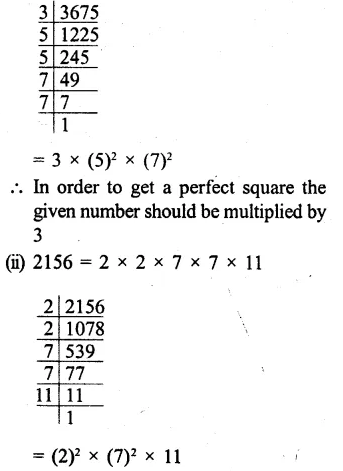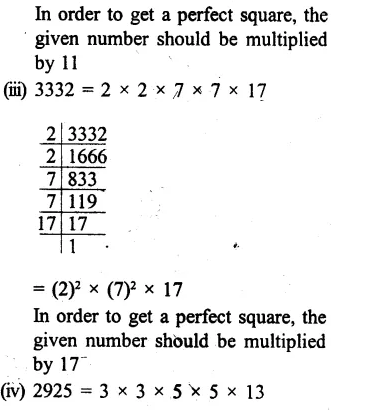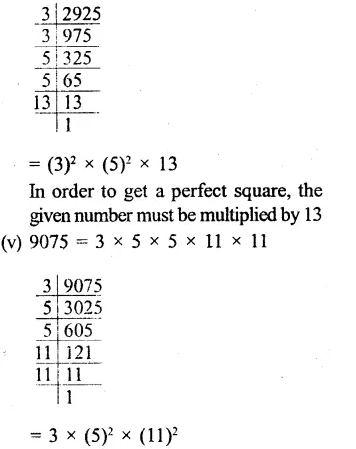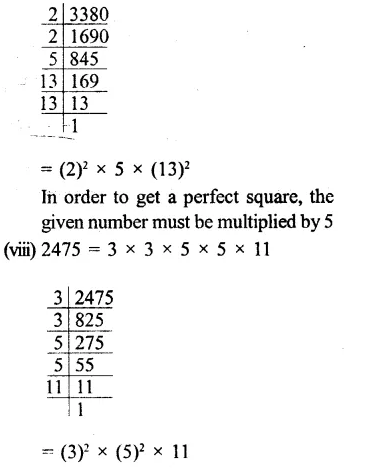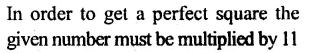Question 4.
Solution:
(i) 1575
= 3 x 3 x 5 x 5 x 7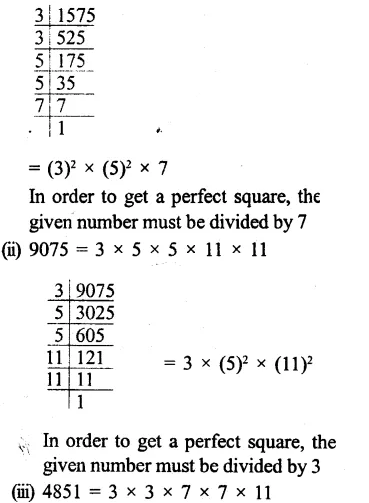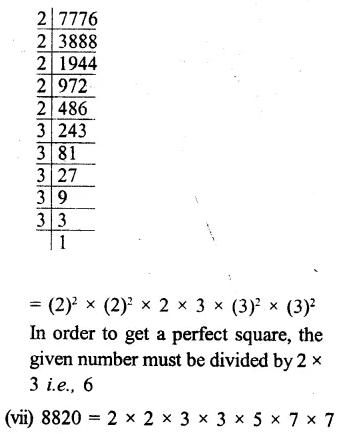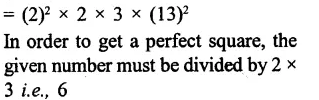Question 5.
Solution:
The largest two digit number = 99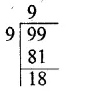Finding the square root of 99, we get remainder = 18
∴The greatest two digit number which is a perfect square will be = 99 – 18 = 81

Question 6.
Solution:
The largest 3 digit number = 999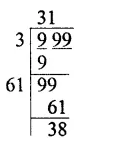Finding the square root of 999, we get remainder = 38
∴The greatest 3 digit number which is a perfect square = 999 – 38 = 961

Hope given RS Aggarwal Solutions Class 8 Chapter 3 Squares and Square Roots Ex 3A are helpful to complete your math homework.

If you have any doubts, please comment below. Learn Insta try to provide online math tutoring for you.